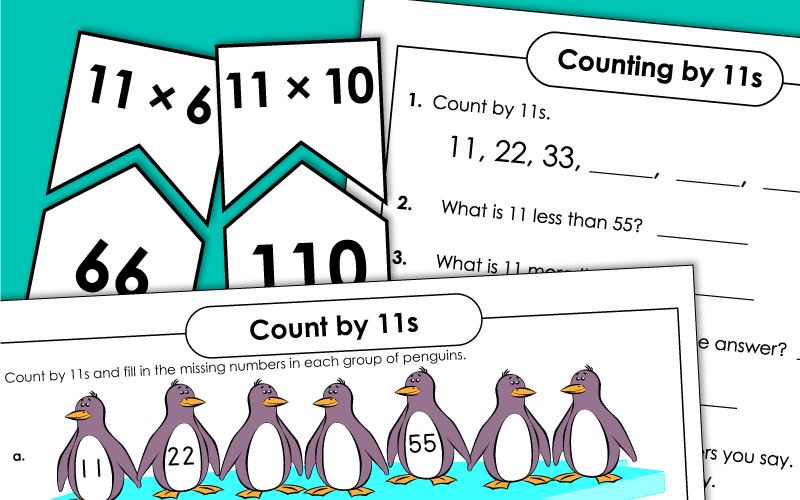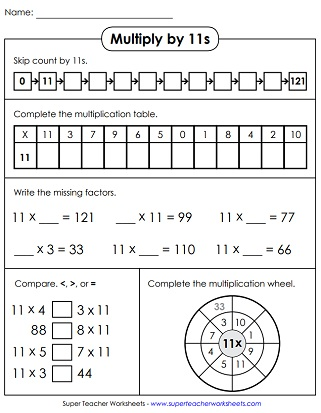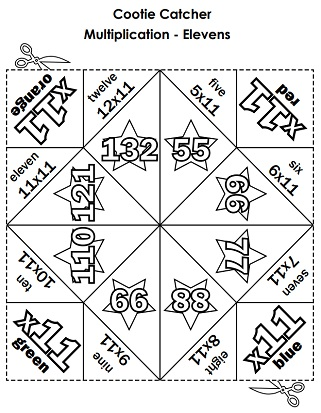# Multiplying by 11

On this page, you'll find worksheets on multiplying by the number 11. Includes drill worksheets, a speed test, and skip counting activities.## Multiplication by11s Only

Complete the multiplication table and the multiplication wheel. Count by 11s and fill in the table.
Fill in the answers to the multiplication problems. All problems have a factor of 11. Then color the picture according to the key.
Students can practice their multiplication facts with 11s and 12s with this printable activity.
In this printable math activity, students will multiply by 11s in order to fill out all the bubbles in the multiplication caterpillar.
Cut, color, and fold this origami cootie catcher. Then use it to practice multiplication faces with factors of eleven.
Use these flash cards to practice multiplication facts with 11 as a factor. File includes: 11 flash cards, 5 super challenge cards, a self-quiz, and a sorting mat.
Use scissors and crayons to build a math slider. Then use it to review basic facts with 11 as a factor.

## Skip Counting by 11s

At the top of this page, practice skip counting by 11s. Fill in the missing numbers. Then answer the skip-counting (and multiplying by 11) questions that follow.
On this music-themed worksheet, students will count by 11s and fill in the empty boxes.
This page has pictures of penguins all lined up in rows. Count by 11s. Write the correct numbers on the penguins.
Count by 11s, and write the missing numbers on the colorful helium balloons.

## All Facts0s through 11s

Here's a multiplication timed quiz with facts going up to 11x12.
Basic Multiplication

Multiplication & Division Fact Families

These worksheets will help students learn how multiplication and division are related.

Properties of Multiplication

Learn about the associative, commutative, and distributive properties of multiplication.

## Sample Worksheet ImagesMy Account
Site Information